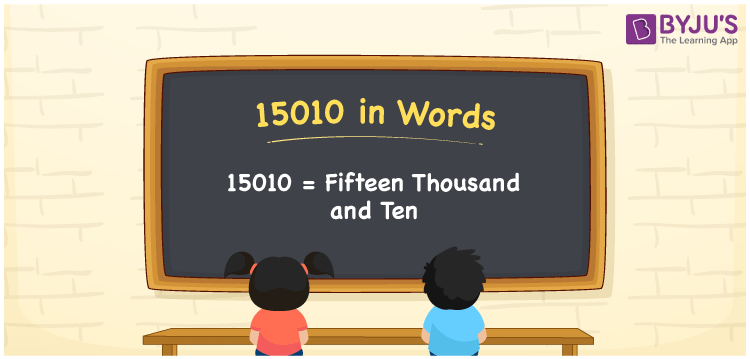# 15010 in Words

We can write 15010 in words as Fifteen thousand and ten. Here, Fifteen thousand and ten is known as the number name or word form of 15010. This number name is used to convey the number verbally to others. If you spent Rs. 15010 for buying a gift; you can say, “I spent Fifteen thousand and ten rupees for buying a gift”. In this article, you will understand how to derive the cardinal number 15010 into words.

 15010 in words Fifteen thousand and ten Fifteen thousand and ten in Numbers 15010

## 15010 in English Words

Generally, we use the English alphabet to express numbers in words. Thus, we spell 15010 in English words as “Fifteen thousand and ten”.## How to Write 15010 in Words?

In this section, you will understand how to write the number 15010 in words with the help of a place value chart given below.

 Ten thousand Thousands Hundreds Tens Ones 1 5 0 1 0

Here, ones = 0, tens = 1, hundreds = 0, thousands = 5, ten thousand = 1

By expanding the above numbers, we get;

1 × Ten thousand + 5 × Thousand + 0 × Hundred + 1 × Ten + 0 × One

= 1 × 10000 + 5 × 1000 + 0 × 100 + 1 × 10 + 0 × 1

= 10000 + 5000 + 10

= Ten Thousand + Five thousand + Ten

= Fifteen thousand + Ten

= Fifteen thousand and ten

Therefore, 15010 in words = Fifteen thousand and ten.

As we know, 15010 is a natural number that is the successor of 15009 and predecessor of 15011.

15010 in words – Fifteen thousand and ten

Is 15010 an odd number? – No

Is 15010 an even number? – Yes

Is 15010 a prime number? – No

Is 15010 a composite number? – Yes

Is 15010 a perfect square number? – No

Is 15010 a perfect cube number? – No

## Frequently Asked Questions on 15010 in Words

Q1

### How do you write 15010 in words?

We can write 15010 in English words as Fifteen thousand and ten.
Q2

### How to write Rs. 15010 in words on a cheque?

On a cheque, we generally write Rs. 15010 in words as “Fifteen thousand and ten rupees only”.
Q3

### What is the word form of 15010?

Fifteen thousand and ten is the word form of the number 15010.